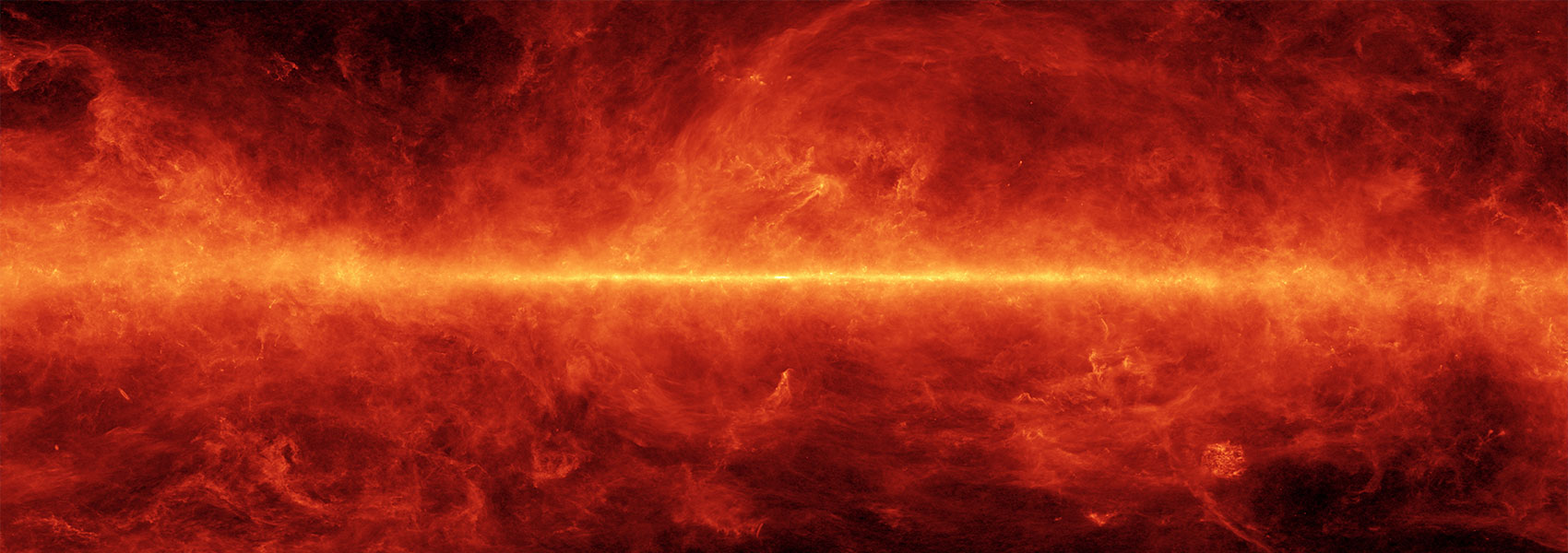# Planetary candidates transiting cool dwarf stars from campaigns 12 to 15 of K2

December 2020 • 2020MNRAS.499.5416C

Authors • Castro González, A. • Díez Alonso, E. • Menéndez Blanco, J. • Livingston, John H. • de Leon, Jerome P. • Suárez Gómez, S. L. • González Gutiérrez, C. • García Riesgo, F. • Bonavera, L. • Iglesias Rodríguez, F. J. • Muñiz, R. • Everett, Mark E. • Scott, N. J. • Howell, Steve B. • Ciardi, David R. • Gonzales, Erica J. • Schlieder, Joshua E. • de Cos Juez, F. J.

Abstract • We analysed the photometry of 20 038 cool stars from campaigns 12, 13, 14, and 15 of the K2 mission in order to detect, characterize, and validate new planetary candidates transiting low-mass stars. We present a catalogue of 25 new periodic transit-like signals in 22 stars, of which we computed the parameters of the stellar host for 19 stars and the planetary parameters for 21 signals. We acquired speckle and AO images, and also inspected archival Pan-STARRS1 images and Gaia DR2 to discard the presence of close stellar companions and to check possible transit dilutions due to nearby stars. False positive probability (FPP) was computed for 22 signals, obtaining FPP < $1{{\ \rm per\ cent}}$ for 17. We consider 12 of them as statistically validated planets. One signal is a false positive and the remaining 12 signals are considered as planet candidates. 20 signals have an orbital period of P $_{\rm orb} \lt 10\,\mathrm{ d}$ , 2 have $10\, \mathrm{ d} \lt$ P $_{\rm orb} \lt 20\, \mathrm{ d}$ , and 3 have P $_{\rm orb} \gt 20\, \mathrm{ d}$ . Regarding radii, 11 candidates and validated planets have computed radius R < 2R, 9 have 2R < R < 4R, and 1 has R > 4R. Two validated planets and two candidates are located in moderately bright stars ( $\rm \mathit{ m}_{kep}\lt 13$ ) and two validated planets and three candidates have derived orbital radius within the habitable zone according to optimistic models. Of special interest is the validated warm super-Earth K2-323 b (EPIC 248616368 b) with T $_{\rm eq} = 318^{+24}_{-43} \, \mathrm{ K}$ , S $_{\rm p} = 1.7\pm 0.2 \, \mathrm{ S}_{\oplus }$ , and R $_{\rm p} = 2.1\pm 0.1 \, \mathrm{ R}_{\oplus }$ , located in an m $\rm _{kep}$ = 14.13 star.